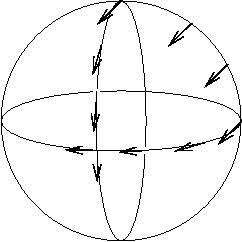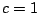Next: Einstein's Equation Up: The Meaning of Einstein's Equation Previous: Introduction

# Preliminaries

Before stating Einstein's equation, we need a little preparation. We assume the reader is somewhat familiar with special relativity -- otherwise general relativity will be too hard. But there are some big differences between special and general relativity, which can cause immense confusion if neglected.

In special relativity, we cannot talk about absolute velocities, but only relative velocities. For example, we cannot sensibly ask if a particle is at rest, only whether it is at rest relative to another. The reason is that in this theory, velocities are described as vectors in 4-dimensional spacetime. Switching to a different inertial coordinate system can change which way these vectors point relative to our coordinate axes, but not whether two of them point the same way.

In general relativity, we cannot even talk about relative velocities, except for two particles at the same point of spacetime -- that is, at the same place at the same instant. The reason is that in general relativity, we take very seriously the notion that a vector is a little arrow sitting at a particular point in spacetime. To compare vectors at different points of spacetime, we must carry one over to the other. The process of carrying a vector along a path without turning or stretching it is called `parallel transport'. When spacetime is curved, the result of parallel transport from one point to another depends on the path taken! In fact, this is the very definition of what it means for spacetime to be curved. Thus it is ambiguous to ask whether two particles have the same velocity vector unless they are at the same point of spacetime.

It is hard to imagine the curvature of 4-dimensional spacetime, but it is easy to see it in a 2-dimensional surface, like a sphere. The sphere fits nicely in 3-dimensional flat Euclidean space, so we can visualize vectors on the sphere as `tangent vectors'. If we parallel transport a tangent vector from the north pole to the equator by going straight down a meridian, we get a different result than if we go down another meridian and then along the equator:Because of this analogy, in general relativity vectors are usually called `tangent vectors'. However, it is important not to take this analogy too seriously. Our curved spacetime need not be embedded in some higher-dimensional flat spacetime for us to understand its curvature, or the concept of tangent vector. The mathematics of tensor calculus is designed to let us handle these concepts `intrinsically' -- i.e., working solely within the 4-dimensional spacetime in which we find ourselves. This is one reason tensor calculus is so important in general relativity.

Now, in special relativity we can think of an inertial coordinate system, or `inertial frame', as being defined by a field of clocks, all at rest relative to each other. In general relativity this makes no sense, since we can only unambiguously define the relative velocity of two clocks if they are at the same location. Thus the concept of inertial frame, so important in special relativity, is banned from general relativity!

If we are willing to put up with limited accuracy, we can still talk about the relative velocity of two particles in the limit where they are very close, since curvature effects will then be very small. In this approximate sense, we can talk about a `local' inertial coordinate system. However, we must remember that this notion only makes perfect sense in the limit where the region of spacetime covered by the coordinate system goes to zero in size.

Einstein's equation can be expressed as a statement about the relative acceleration of very close test particles in free fall. Let us clarify these terms a bit. A `test particle' is an idealized point particle with energy and momentum so small that its effects on spacetime curvature are negligible. A particle is said to be in `free fall' when its motion is affected by no forces except gravity. In general relativity, a test particle in free fall will trace out a `geodesic'. This means that its velocity vector is parallel transported along the curve it traces out in spacetime. A geodesic is the closest thing there is to a straight line in curved spacetime.

Again, all this is easier to visualize in 2d space rather than 4d spacetime. A person walking on a sphere `following their nose' will trace out a geodesic -- that is, a great circle. Suppose two people stand side-by-side on the equator and start walking north, both following geodesics. Though they start out walking parallel to each other, the distance between them will gradually start to shrink, until finally they bump into each other at the north pole. If they didn't understand the curved geometry of the sphere, they might think a `force' was pulling them together.

Similarly, in general relativity gravity is not really a `force', but just a manifestation of the curvature of spacetime. Note: not the curvature of space, but of spacetime. The distinction is crucial. If you toss a ball, it follows a parabolic path. This is far from being a geodesic in space: space is curved by the Earth's gravitational field, but it is certainly not so curved as all that! The point is that while the ball moves a short distance in space, it moves an enormous distance in time, since one second equals about 300,000 kilometers in units where. This allows a slight amount of spacetime curvature to have a noticeable effect.

Next: Einstein's Equation Up: The Meaning of Einstein's Equation Previous: Introduction

© 2006 John Baez and Emory Bunn